# 26. Introduction to Text Classification

## Introduction

Document classification/categorization is a topic in information science, a science dealing with the collection, analysis, classification, categorization, manipulation, retrieval, storage and propagation of information.

This might sound very abstract, but there are lots of situations nowadays, where companies are in need of automatic classification or categorization of documents.Just think about a large company with thousands of incoming mail pieces per day, both electronic or paper based. Lot's of these mail pieces are without specific addressee names or departments. Somebody has to read these texts and has to decide what kind of a letter it is ("change of address", "complaints letter", "inquiry about products", and so on) and to whom the document should be proceeded. This "somebody" can be an automated text classification system.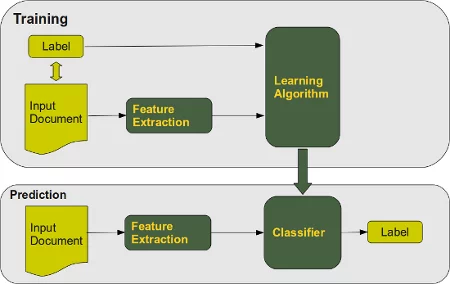Automated text classification, also called categorization of texts, has a history, which dates back to the beginning of the 1960s. But the incredible increase in available online documents in the last two decades, due to the expanding internet, has intensified and renewed the interest in automated document classification and data mining. In the beginning text classification focussed on heuristic methods, i.e. solving the task by applying a set of rules based on expert knowledge. This approach proved to be highly inefficient, so nowadays the focus has turned to fully automatic learning and clustering methods.

The task of text classification consists in assigning a document to one or more categories, based on the semantic content of the document. Document (or text) classification runs in two modes:

• The training phase and the
• prediction (or classification) phase.

The training phase can be divided into three kinds:

• supervised document classification is performed by an external mechanism, usually human feedback, which provides the necessary information for the correct classification of documents,
• semi-supervised document classification, a mixture between supervised and unsupervised classification: some documents or parts of documents are labelled by external assistance,
• unsupervised document classification is entirely executed without reference to external information.

We will implement a text classifier in Python using Naive Bayes. Naive Bayes is the most commonly used text classifier and it is the focus of research in text classification. A Naive Bayes classifier is based on the application of Bayes' theorem with strong independence assumptions. "Strong independence" means: the presence or absence of a particular feature of a class is unrelated to the presence or absence of any other feature. Naive Bayes is well suited for multiclass text classification.

Live Python training

Enjoying this page? We offer live Python training courses covering the content of this site.

Enrol here

## Formal Definition

Let C = { c1, c2, ... cm} be a set of categories (classes) and D = { d1, d2, ... dn} a set of documents.

The task of the text classification consists in assigning to each pair ( ci, dj ) of C x D (with 1 ≤ i ≤ m and 1 ≤ j ≤ n) a value of 0 or 1, i.e. the value 0, if the document dj doesn't belong to ci

This mapping is sometimes referred to as the decision matrix:

$d_1$ ... $d_j$ ... $d_n$
$c_1$ $a_{11}$ ... $a_{1j}$ ... $a_{1n}$
... ... ... ... ... ...
$c_i$ $a_{i1}$ ... $a_{ij}$ ... $a_{in}$
... ... ... ... ... ...
$c_m$ $a_{m1}$ ... $a_{mj}$ ... $a_{mn}$

The main approaches to solve this task are:

• Naive Bayes
• Support Vector Machine
• Nearest Neighbour

## Naive Bayes Classifier

A Bayes classifier is a simple probabilistic classifier based on applying Bayes' theorem with strong (naïve) independence assumptions, i.e. an "independent feature model". In other words: A naive Bayes classifier assumes that the presence (or absence) of a particular feature of a class is unrelated to the presence (or absence) of any other feature.

### Formal Derivation of the Naive Bayes Classifier: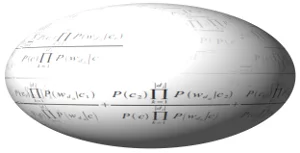Let C = { c1, c2, ... cm} be a set of classes or categories and D = { d1, d2, ... dn} be a set of documents.
Each document is labeled with a class.
The set D of documents is used to train the classifier.
Classification consists in selecting the most probable class for an unknown document.

The number of times a word wt occurs within a document di will be denoted as Nit. NtC denotes the number of times a word wt ocurs in all documents of a given class C.
P(di|cj) is 1, if di is labelled as cj, 0 otherwise

The probability for a word $w_t$ given a class $c_j$: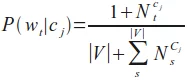The probability for a class cj is the quotient of the number of Documents of cj and the number of documents of all classes, i.e. the learn set: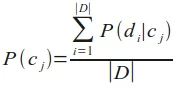Finally, we come to the formula we need to classify an unknown document, i.e. the probability for a class cj given a document di: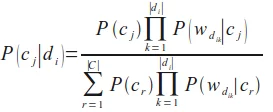Unfortunately, the formula of P(c|di) we have just given is numerically not stable, because the denominator can be zero due to rounding errors. We change this by calculating the reciprocal and reformulate the expression as a sum of stable quotients: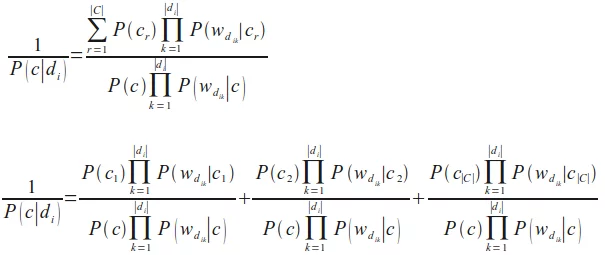We can rewrite the previous formula into the following form, our final Naive Bayes classification formula, the one we will use in our Python implementation in the following chapter: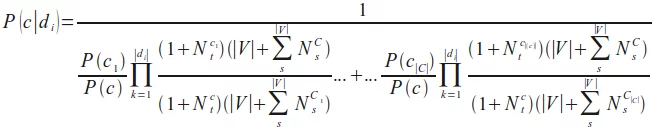Live Python training

Enjoying this page? We offer live Python training courses covering the content of this site.

Upcoming online Courses

Enrol here

There are lots of articles on text classification. We just name a few, which we have used for our work:

• Fabrizio Sebastiani. A tutorial on automated text categorisation. In Analia Amandi and Alejandro Zunino (eds.), Proceedings of the 1st Argentinian Symposium on Artificial Intelligence (ASAI'99), Buenos Aires, AR, 1999, pp. 7-35.
• Lewis, David D., Naive (Bayes) at Forty: The independence assumption in informal retrieval, Lecture Notes in Computer Science (1998), 1398, Issue: 1398, Publisher: Springer, Pages: 4-15
• K. Nigam, A. McCallum, S. Thrun and T. Mitchell, Text classification from labeled and unlabeled documents using EM, Machine Learning 39 (2000) (2/3), pp. 103-134.

Live Python training

Enjoying this page? We offer live Python training courses covering the content of this site.

Upcoming online Courses

Enrol here